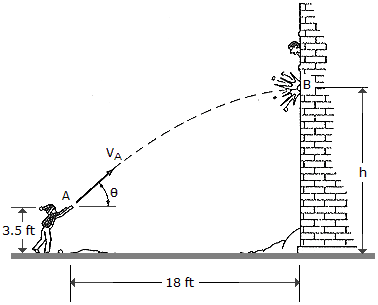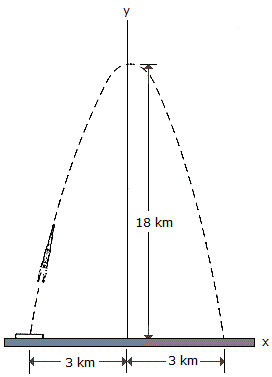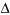# Engineering Mechanics - Kinematics of Particle (KOP)

### Exercise :: Kinematics of Particle (KOP) - General Questions

• Kinematics of Particle (KOP) - General Questions
31.The boy throws a snowball such that it strikes the wall of the building at the maximum height of its trajectory. If it takes t = 1.5 s to travel from A to B, determine the velocity vA at which it was thrown, the angle of release, and the height h.

 A. vA = 12.00 ft/s,= 24.4E, h = 21.7 ft B. vA = 49.8 ft/s,= 76.0E, h = 39.7 ft C. vA = 36.3 ft/s,= 24.4E, h = 18.2 ft D. vA = 48.3 ft/s,= 65.6E, h = 39.7 ft

Explanation:

No answer description available for this question. Let us discuss.

32.For a short time the missile moves along the parabolic path y = (18 - 2x2) km. If motion along the ground is measured as x = (4t - 3) km, where t is in seconds, determine the magnitudes of the missile's velocity and acceleration when t = 1 s.

 A. v = 5.66 km/s, a = 4.0 km/s2 B. v = 16.49 km/s, a = 64.0 km/s2 C. v = 16.00 km/s, a = 22.6 km/s2 D. v = 4.00 km/s, a = 16.03 km/s2

Explanation:

No answer description available for this question. Let us discuss.

33.

A small metal particle passes downward through a fluid medium while being subjected to the attraction of a magnetic field such that its position is observed to be s = (15t3 - 3t) mm, where t is measured in seconds. Determine (a) the particle's displacement from t = 2 s to t = 4 s, and (b) the velocity and acceleration of the particle when t = 5 s.

 A.s = 834 mm, v = 1122 mm/s, a = 450 mm/s2 B.s = 114 mm, v = 1122 mm/s, a = 450 mm/s2 C.s = 114 mm, v = 450 mm/s, a = 1122 mm/s2 D.s = 834 mm, v = 450 mm/s, a = 1122 mm/s2

Explanation:

No answer description available for this question. Let us discuss.

34.

A car is traveling at a speed of 80 ft/s when the brakes are suddenly applied, causing a constant deceleration of 10 ft/s2. Determine the time required to stop the car and the distance traveled before stopping.

 A. t = 8 s, s = 800 ft B. t = 8 s, s = 320 ft C. t = 4 s, s = 240 ft D. t = 4 s, s = 40 ft